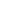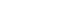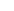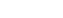# Autoencoders with Deep Learning(AI)

0
245## Introduction Autoencoders with Deep Learning

The nature of the Autoencoders with Deep Learning is to encode information, to compress it. Working or Research on computer vision or any deep learning project produced a huge amount of data. So, it’s difficult when transferring the data along with all input and output. Data compression is a big problematic topic that’s used in computer vision. A computer network, natural language processing, and much more deep learning projects.

Data compression is to convert our input into a smaller representation that we recreate to a degree of quality.

For example, consider a ZIP file. When we create a ZIP file, we compress our files so that they take up fewer bytes. Then share the ZIP file.  After that you want to access the content, we can uncompress.

Trying to discuss deep learning-based anomaly detection without prior context on what autoencoders are and how they work would be challenging to follow, comprehend, and digest.

## Autoencoders with Deep Learning

In our example from the image above, the encoded information is localized in the middle layer, which is sometimes called the code. This is the most interesting information for us. The first part of Autoencoders is called encoding and can be represented with the function – f(x), where x is input information.

The code is the result of the encoding and can be represented like this – h = f(x). Finally, the part of the architecture after the middle layer is called decryption, and it produces the reconstruction of the data – y = g(h) = g(f(x)). To sum it up, the Autoencoder receives input data, encodes it in the middle layer and then returns the same data on the output layer. Sometimes the middle layer can have more neurons than we have in input and output layers. Then we are dealing with Overcomplete Autoencoders.

## Definition

Autoencoders are a type of ‘unsupervised neural network’. In another words no class label or no target feature that seek to:

1. Accept as input set of data.
2.  Internally compress the input data into a latent-space representation.
3. Reconstruct the input data from this latent representation(i.e. output)

An autoencoder does two tasks, it encodes an image and then decodes it.

Widget not in any sidebars

## Encode

An input image is taken and through a series of convolutional neural networks, the size of the image is compressed into a small vector. This compress vector represent the features of the image from which another image can be reconstructed

If we denote our input data asand the encoder as, then the output latent-space representation,, would be.

## Decode

From the compressed vector, we apply a series of deconvolution layers which blows up the size of the image and restores it back to its original size.

If we denote the decoder function asand the output of the detector as, then we can represent the decoder as.

Using our mathematical notation, the entire training process of the autoencoder can be written as:

## Use of an  Autoencoders with Deep Learning

Autoencoders  can be used to remove the error (i.e. noise), perform image color, try to match the edges of the image, and various related images. If the input image is noisy or any distraction to the autoencoder, they provide a denoised image it means reduce error.

The autoencoder will try to denoise the image by learning the latent features of the image and using that to reconstruct an image without noise. The reconstruction error can be calculated as a measure of distance between the pixel values of the output image and ground truth image.

Here you can see that:

• We input a digit to the autoencoder.
• The encoder subnetwork creates a latent representation of the digit. This latent representation is substantially smaller (in terms of dimensionality) than the input.
• The decoder subnetwork then reconstructs the original digit from the latent representation.

### An autoencoder reconstructs it’s input — so what’s the big deal?

Now some questions arise.

If the goal of an autoencoder is just to reconstruct the input, why even use the network in the first place?

Yes, during the training process, our goal is to train a network that can learn how to reconstruct our input data — but the true value of the autoencoder lives inside that latent-space representation.

Keep in mind that autoencoders compress our input data and, more to the point, when we train autoencoders, what we really care about is the encoder,, and the latent-space representation,.

The decoder,, is used to train the autoencoder end-to-end, but in practical applications, we often (but not always) care more about the encoder and the latent-space.

Later in this tutorial, we’ll be training an autoencoder on the MNIST dataset. The MNIST dataset consists of digits that are 28×28 pixels with a single channel, implying that each digit is represented by 28 x 28 = 784 values. The autoencoder we’ll be training here will be able to compress those digits into a vector of only 16 values — that’s a reduction of nearly 98%!

So what can we do if an input data point is compressed into such a small vector?

That’s where things get really interesting.

## Application

Autoencoders are typically used for:

• Dimensionality reduction (i.e., think PCA but more powerful/intelligent).
• Denoising (ex., removing noise and preprocessing images to improve OCR accuracy).
• Anomaly/outlier detection (ex., detecting mislabeled data points in a dataset or detecting when an input data point falls well outside our typical data distribution).

Outside of the computer vision field, you’ll see autoencoders applied to Natural Language Processing (NLP) and text comprehension problems, including understanding the semantic meaning of words, constructing word embeddings, and even text summarization.

Widget not in any sidebars

## Tutorial

I will share a note book file. Just paste it.

## Conclusion

In this blog, understand about the reduction of error edge to edge and try to get better output.

This is good for, but even though autoencoders might struggle to keep up with GANs. GAN(Generative Adversarial Network) they are highly efficient in certain tasks such as anomaly detection and others.